@Team 2019-02-14T12:10:45.000000Z 字数 15314 阅读 1687

# 深入理解one-stage目标检测算法（下篇）

叶虎

## 数据

VOC数据集包含图像和不同任务的标注，这里我们仅关注目标检测的标注，共有20个类别：

aeroplane    bicycle  bird   boat       bottle
bus          car      cat    chair      cow
diningtable  dog      horse  motorbike  person
pottedplant  sheep    sofa   train      tvmonitor


VOC数据集附带一个建议的训练/验证集分割，大约为50/50。由于数据集不太大，因此将50%的数据用于验证似乎有点浪费。因此，通常将训练集和验证集组合成一个大的训练集“trainval”（总共16551张图像），然后随机选取10%左右的图像用于验证。可以在2007测试集上测试模型，因为label已经给出。还有一个2012年的测试集，但label是不公开的（也有习惯于将2007年测试集包括在训练数据中，数据越多越好）。

2007+2012组合训练集有8218个带物体框标注的图像，验证集有8333个图像，2007测试集有4952个图像。这比ImageNet的130万张图片要少得多，所以最好使用迁移学习，而不是从头开始训练模型。这就是为什么我们从一个已经在ImageNet上预训练过的特征提取器开始。

<annotation>
<folder>VOC2007</folder>
<filename>003585.jpg</filename>
<source>
<database>The VOC2007 Database</database>
<annotation>PASCAL VOC2007</annotation>
<image>flickr</image>
<flickrid>304100796</flickrid>
</source>
<owner>
<flickrid>Huw Lambert</flickrid>
<name>huw lambert</name>
</owner>
<size>
<width>333</width>
<height>500</height>
<depth>3</depth>
</size>
<object>
<name>person</name>
<truncated>0</truncated>
<difficult>0</difficult>
<bndbox>
<xmin>138</xmin>
<ymin>183</ymin>
<xmax>259</xmax>
<ymax>411</ymax>
</bndbox>
</object>
<object>
<name>motorbike</name>
<truncated>0</truncated>
<difficult>0</difficult>
<bndbox>
<xmin>89</xmin>
<ymin>244</ymin>
<xmax>291</xmax>
<ymax>425</ymax>
</bndbox>
</object>
</annotation>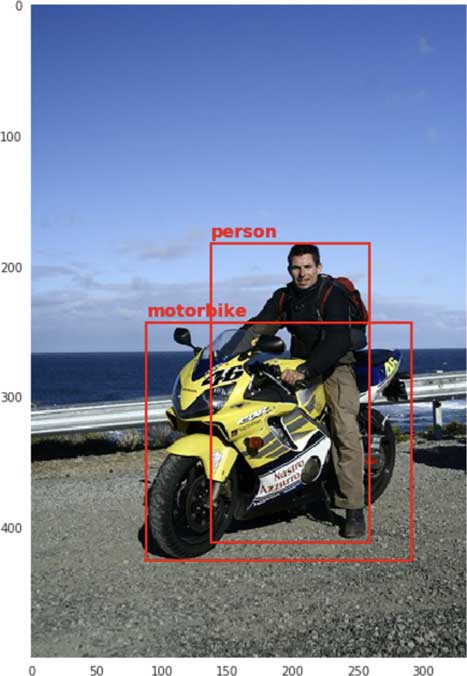VOC2007和2012共包含如下的图像：

dataset   images    objects
------------------------------
train     8218      19910
val       8333      20148
test      4952      12032     (2007 only)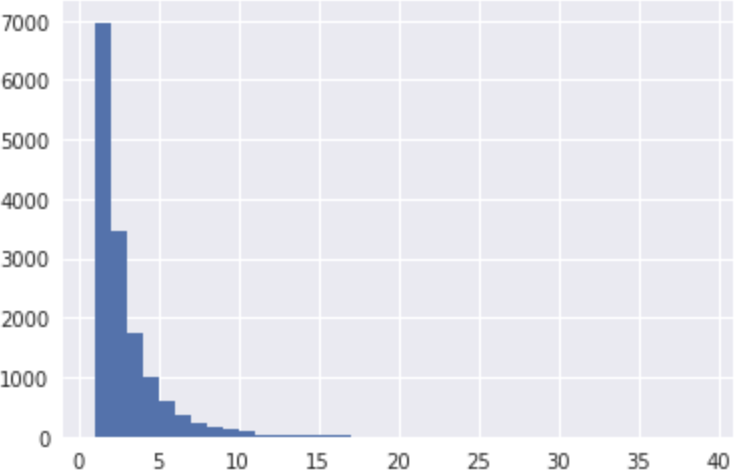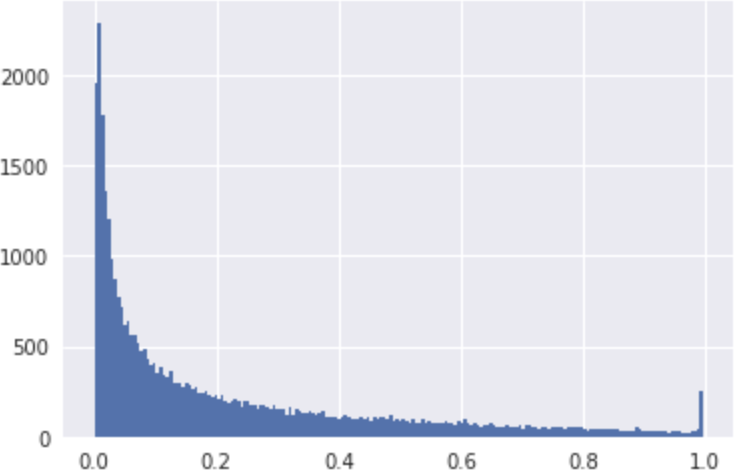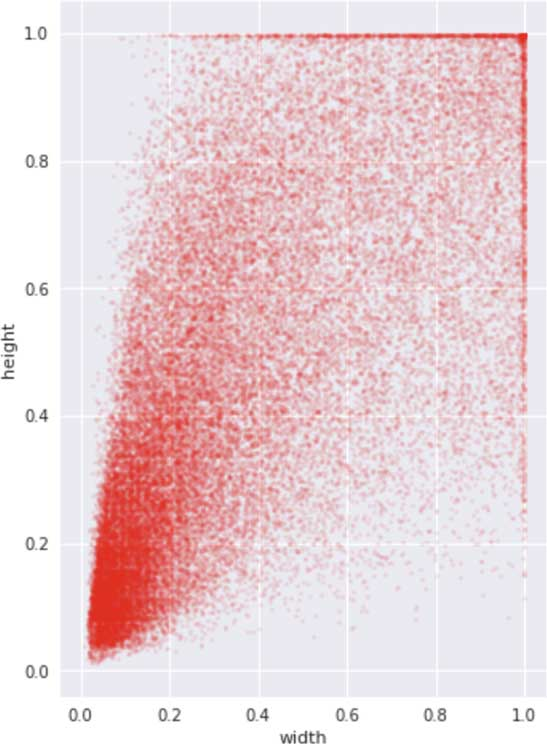YOLO的数据扩增流程如下：

• 加载原始图像；
• 通过随机增加/减去原始大小的20%来选择新的宽度和高度；
• 按照新大小裁剪图像，如果新图像在一个或多个边上大于原始图像，则用零填充
• 将图像resize到416×416，使其成为正方形
• 随机水平翻转图像（50%的概率）
• 随机改变图像的色调、饱和度和曝光（亮度）
• 对应地，要通过移动和缩放边界框坐标来调整边界框，以适应前面所做的裁剪和调整大小，以及水平翻转等操作

• 随机裁剪一个图像区域，使该区域中物体的最小IOU（与原始图像中物体）为0.1、0.3、0.5、0.7或0.9，IOU越小，模型就越难检测到物体
• 使用“缩小”增强，将图像变小，从而构建包含小物体的训练数据，这对于模型更好地处理小物体很有用。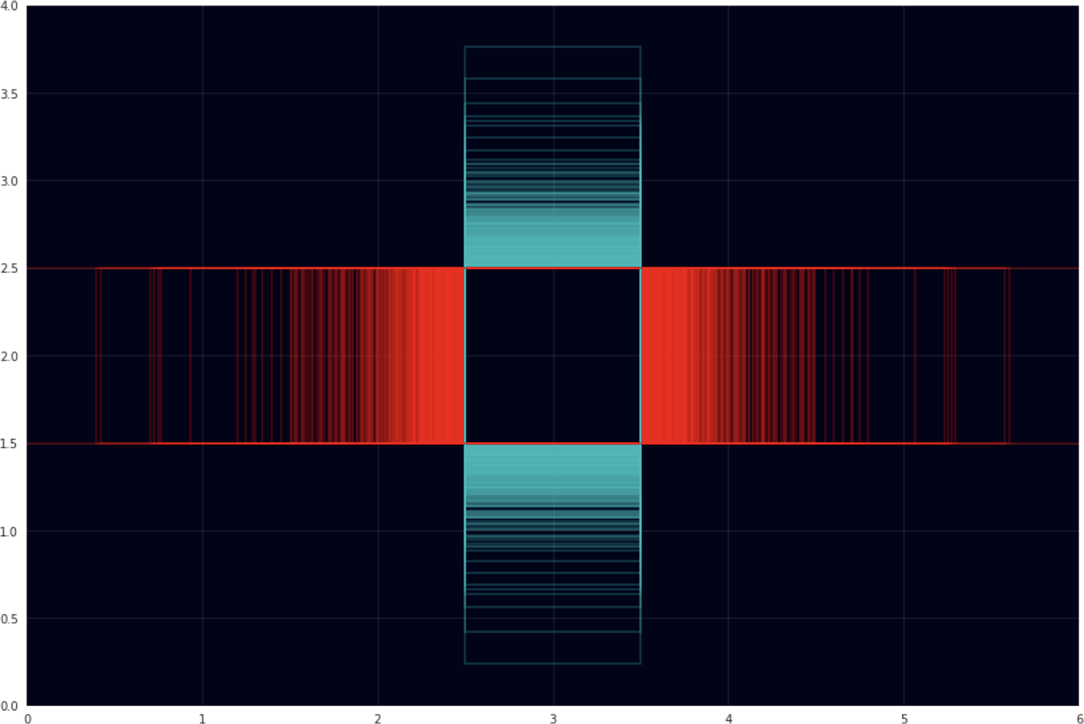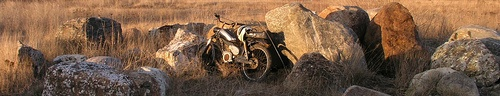- 直接将图像resize到416×416，这可能会挤压图像；
- 将最小边调整为416，然后从图像中裁剪出416×416区域；
- 将最大边调整为416，用零填充另外的短边；

## 模型是如何训练的

VOC数据集给出的边界框标注为xmin, ymin, xmax, ymax。由于我们需要知道边界框中心，所以需要将边界框坐标转为center x, center y, width, and height。我们一般会先将边界框坐标归一化到[0, 1]，这样它们独立于输入图像的大小（因为训练图像的大小并不一致）。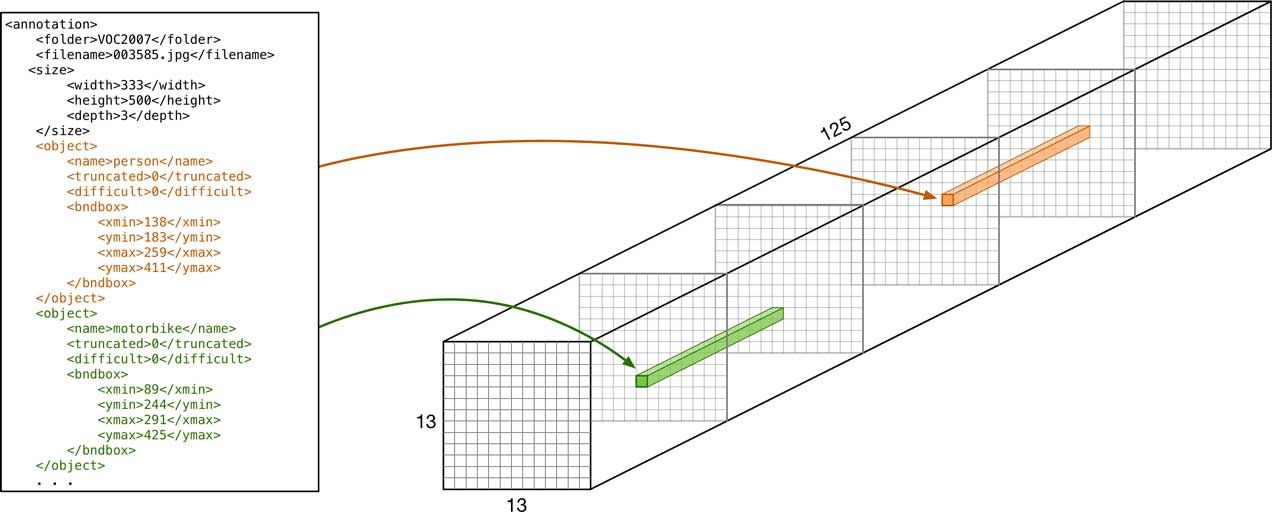YOLO的解决方案比较粗暴：每次随机打乱真实框，每个单元只选择第一个进入它中心的物体。因此，如果一个新的真实框与一个已经负责另一个物体的单元相匹配，那么我们就只能忽略它了。这意味着在YOLO中，每个单元至多有一个检测器被匹配到物体，而其他检测器不应该检测到任何东西（如果检测到了，就会受到惩罚）。

## 损失函数

• 这个检测器没有与之相关的真实框，这是负例，它不应该检测到任何物体（即它应该预测一个置信度为0的边界框）。
• 这个检测器匹配到了一个真实框，即正例，它负责检测到物体。

• 当坐标错误
• 当置信度太低时
• 分类错误

SSD、YOLO、Squezedet、Detectnet和其他one-stage目标检测模型的损失函数可能有差异，但是它们往往由相同的部分组成。

（1）没有被匹配的检测器（负例）

no_object_loss[i, j, b] = no_object_scale * (0 - sigmoid(pred_conf[i, j, b]))**2


SSD没有这项loss，因为它将背景类看成一个特殊类进行处理。如果预测的是背景类，那个检测器被认为没有检测到物体。

（2）被匹配的检测器（正例）

（a）置信度

object_loss[i, j, b] = object_scale * (1 - sigmoid(pred_conf[i, j, b]))**2


object_loss[i, j, b] = object_scale *
(IOU(truth_coords, pred_coords) - sigmoid(pred_conf[i, j, b]))**2


（b）类别概率

YOLOv1和v2按如下方式计算分类的loss:

class_loss[i, j, b] = class_scale * (true_class - softmax(pred_class))**2


（c）边界框坐标

coord_loss[i, j, b] = coord_scale * ((true_x[i, j, b] - pred_x[i, j, b])**2                                   + (true_y[i, j, b] - pred_y[i, j, b])**2                                   + (true_w[i, j, b] - pred_w[i, j, b])**2                                   + (true_h[i, j, b] - pred_h[i, j, b])**2)

box_x[i, j, b] = (i + sigmoid(pred_x[i, j, b])) * 32box_y[i, j, b] = (j + sigmoid(pred_y[i, j, b])) * 32box_w[i, j, b] = anchor_w[b] * exp(pred_w[i, j, b]) * 32box_h[i, j, b] = anchor_h[b] * exp(pred_h[i, j, b]) * 32

true_x[i, j, b] = ground_truth.center_x - grid[i, j].center_xtrue_y[i, j, b] = ground_truth.center_y - grid[i, j].center_ytrue_w[i, j, b] = log(ground_truth.width / anchor_w[b])true_h[i, j, b] = log(ground_truth.height / anchor_h[b])

difference = abs(true_x[i, j, b] - pred_x[i, j, b])if difference < 1:    coord_loss_x[i, j, b] = 0.5 * difference**2else:    coord_loss_x[i, j, b] = difference - 0.5

• 一个包含图片以及边界框标注的数据集（如Pascal VOC）；
• 一个可以拥有网格检测器的模型，并采用一个匹配策略将真实框转化为目标张量；
• 一个计算预测值与目标值的损失函数。

for i in 0 to 12:  for j in 0 to 12:    for b in 0 to 4:      gt = target[i, j, b]   # ground-truth      pred = grid[i, j, b]   # prediction from model      # is this detector responsible for an object?      if gt.conf == 1:        iou = IOU(gt.coords, pred.coords)        object_loss[i, j, b] = (iou - sigmoid(pred.conf[i, j, b]))**2        coord_loss[i, j, b] = sum((gt.coords - pred.coords)**2)        class_loss[i, j, b] = cross_entropy(gt.class, pred.class)      else:        no_object_loss[i, j, b] = (0 - sigmoid(pred.conf[i, j, b]))**2

loss = no_object_scale * sum(no_object_loss) +           object_scale * sum(object_loss) +            coord_scale * sum(coord_loss) +            class_scale * sum(class_loss)

# the mask is 1 for detectors that have an object, 0 otherwisemask = (target.conf == 1)# compute IOUs between each detector's predicted box and# the corresponding ground-truth box from the target tensorious = IOU(target.coords, grid.coords)# compute the loss terms for the entire grid at once:object_loss = sum(mask * (ious - sigmoid(grid.conf))**2)coord_loss = sum(mask * (target.coords - grid.coords)**2)class_loss = sum(mask * (target.class - softmax(grid.class))**2)no_object_loss = sum((1 - mask) * (0 - sigmoid(grid.conf))**2)

• 多尺度训练。一般情况下，目标检测模型用于不同大小的图片，因而也包含不同尺度的物体。一个可以让模型可以对不同大小的输入泛化的方法是每一定的迭代过程中随机选择不同的输入尺寸。比如随机从320×320到608×608之间选择的输入，而不是恒定在416x416。
• 热身训练（Warm-up training）。 YOLO在早期训练阶段为每个单元中心增加一个假的真实框（先验框），采用这个额外的坐标损失来鼓励模型的预测可以匹配到检测器的先验框。
• 难例挖掘（Hard negative mining）。前面已经说过大部分检测器是不负责检测任何物体的。这意味着正例数量要远少于负例。YOLO采用一个超参数no_object_scale 来处理这种情况，但是SSD采用难例挖掘：它不是计算所有负例的损失，而是只计算那些预测结果最错的部分损失（即置信度较高的负例）。

## 如何评价模型

• 每个检测物体的分类准确度；
• 预测框与真实框的重合度（IOU）
• 模型是否找到图片中的所有物体（召回，recall）。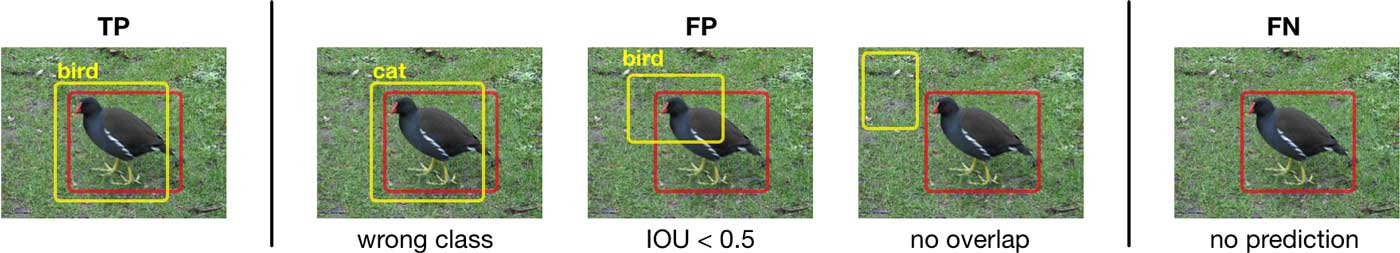precision = TP / (TP + FP)

recall = TP / (TP + FN)

recall和precision的唯一区别是分母不同，前者是真阳数加假阴数，即所有真实物体的总数。对于假阴，就是检测器没有找到一个真实的物体，或者给出的置信度较低。

sort the predictions by confidence score (high to low)for each prediction:    true_boxes = get the annotations with same class as the prediction                     and that are not marked as "difficult"    find IOUs between true_boxes and prediction    choose ground-truth box with biggest IOU overlap    if biggest IOU > threshold (which is 0.5 for Pascal VOC):        if we do not already have a detection for this ground-truth box:            TP += 1        else:            FP += 1    else:        FP += 1

precision-recall曲线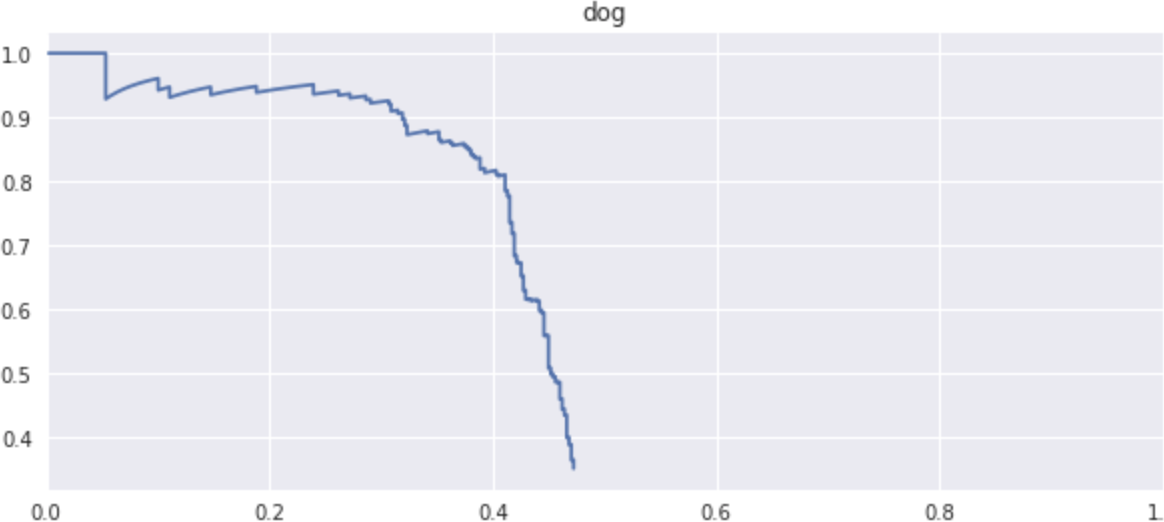• 私有
• 公开
• 删除